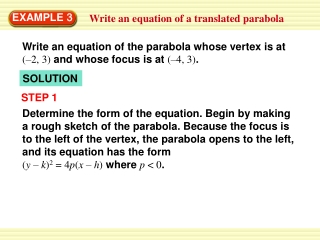DownloadDownload PresentationEXAMPLE 3

# EXAMPLE 3

Télécharger la présentation## EXAMPLE 3

- - - - - - - - - - - - - - - - - - - - - - - - - - - E N D - - - - - - - - - - - - - - - - - - - - - - - - - - -
##### Presentation Transcript

1. EXAMPLE 3 Write an equation of a translated parabola Write an equation of the parabola whose vertex is at (–2, 3) and whose focus is at (–4, 3). SOLUTION STEP 1 Determine the form of the equation. Begin by making a rough sketch of the parabola. Because the focus is to the left of the vertex, the parabola opens to the left, and its equation has the form (y – k)2 = 4p(x – h) where p < 0.

2. EXAMPLE 3 Write an equation of a translated parabola STEP 2 Identify h and k. The vertex is at (–2, 3), so h = –2 and k = 3. STEP 3 Find p. The vertex (–2, 3)and focus (–4, 3)both lie on the line y = 3, so the distance between them is | p | = | –4 – (–2) | = 2, and thus p = +2. Because p < 0, it follows that p = –2, so 4p = –8.

3. EXAMPLE 3 Write an equation of a translated parabola ANSWER The standard form of the equation is (y – 3)2 = –8(x + 2).

4. (x – h)2 (y – k)2 + = 1 a2 b2 EXAMPLE 4 Write an equation of a translated ellipse Write an equation of the ellipse with foci at (1, 2) and (7, 2) and co-vertices at (4, 0) and (4, 4). SOLUTION STEP 1 Determine the form of the equation. First sketch the ellipse. The foci lie on the major axis, so the axis is horizontal. The equation has this form:

5. ) ( 1 + 7 2 + 2 (h, k) = , 2 2 EXAMPLE 4 Write an equation of a translated ellipse STEP 2 Identify hand kby finding the center, which is halfway between the foci (or the co-vertices) = (4, 2) STEP 3 Find b, the distance between a co-vertex and the center (4, 2), and c, the distance between a focus and the center. Choose the co-vertex (4, 4) and the focus (1, 2): b= | 4 – 2 | = 2and c= | 1 – 4 | = 3.

6. Find a. For an ellipse, a2 = b2 + c2 = 22 + 32 = 13,soa = 13 ANSWER The standard form of the equation is + = 1 (x – 4)2 (y – 2)2 13 4 EXAMPLE 4 Write an equation of a translated ellipse STEP 4

7. EXAMPLE 5 Identify symmetries of conic sections Identify the line(s) of symmetry for each conic section in Examples 1 – 4. SOLUTION For the hyperbola in Example 2, x = –1 and y = 3 are lines of symmetry For the circle in Example 1, any line through the center (2, –3) is a line of symmetry.

8. EXAMPLE 5 Identify symmetries of conic sections For the ellipse in Example 4, x = 4 and y = 2 are lines of symmetry. For the parabola in Example 3, y = 3 is a line of symmetry.

9. ANSWER The standard form of the equation is – = 1 ANSWER (x + 4)2 (y – 3)2 The standard form of the equation is (x – 3)2 = 12(y + 1). 9 16 for Examples 3, 4 and 5 GUIDED PRACTICE 5. Write the equation ofparabola with vertex at (3, – 1) and focus at (3, 2). 6. Write the equation of the hyperbola with vertices at (–7,3) and (–1, 3) and foci at (–9, 3) and (1, 3).

10. ANSWER x = 5 and y = 0. (x – 5)2 (y)2 64 16 for Examples 3, 4 and 5 GUIDED PRACTICE Identify the line(s) of symmetry for the conic section. 7. + = 1

11. ANSWER x = –5 for Examples 3, 4 and 5 GUIDED PRACTICE Identify the line(s) of symmetry for the conic section. (x + 5)2 = 8(y – 2). 8.

12. ANSWER x = 1 and y = 2. (x – 1)2 49 for Examples 3, 4 and 5 GUIDED PRACTICE Identify the line(s) of symmetry for the conic section. 9. (y – 2)2 – = 1 121